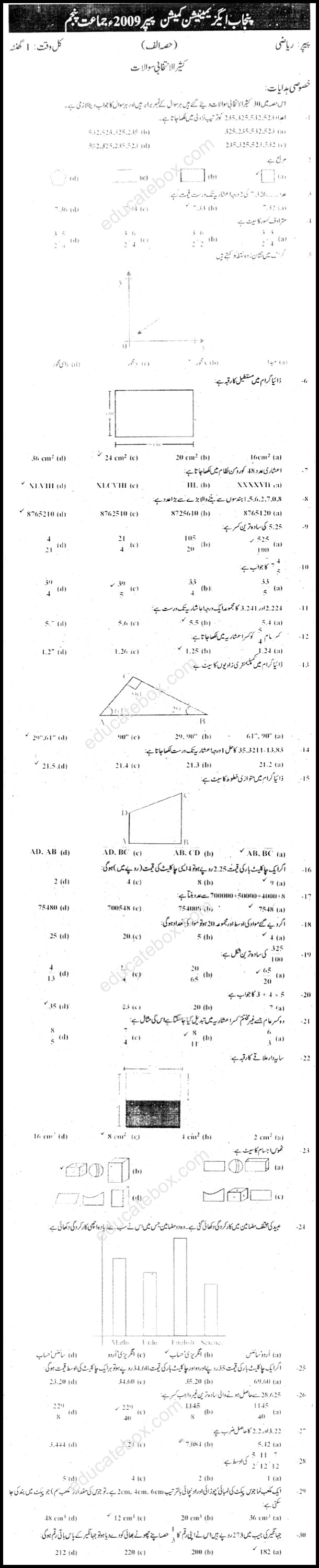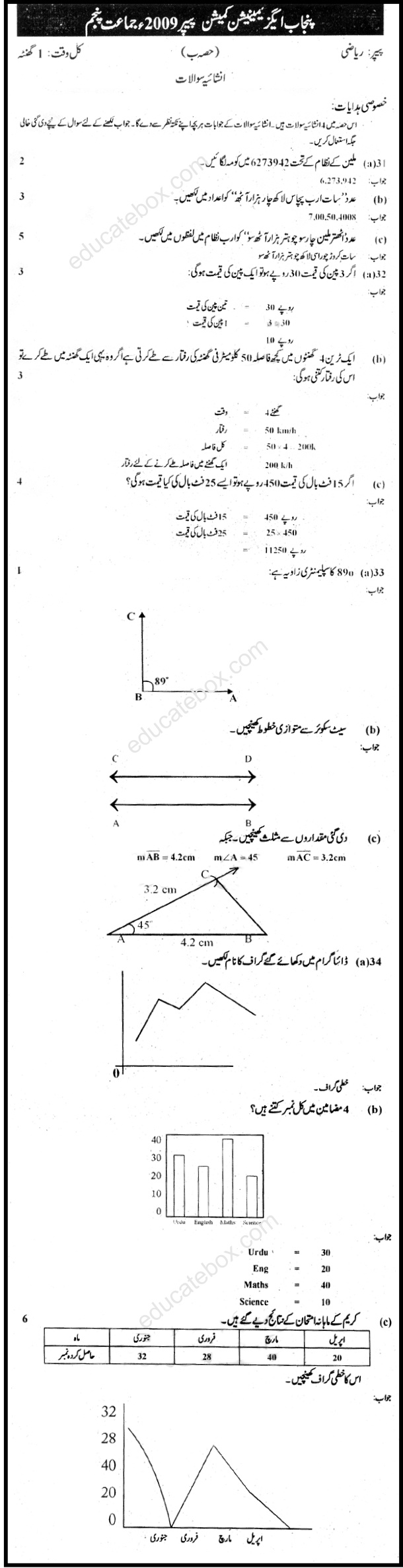# Past Paper Class 5 Maths Punjab Education Commission 2009 Solved Paper

0
784

Class 5 Urdu Medium Maths paper of 2009 has following multiple choice questions:

• Write numbers 235,325,532,525 in ascending order?
• What is the shape of rectangle?
• What is area of rectangle?
• Write decimal number 48 in Roman Number System?
• What is the greatest number formed by digits 1,5,6,2,7,0,8?
• What is lowest form of 5.25?
• What is addition of 3.241 and 2.224 up to 1 decimal place? The answer is 5.5.
• Find set of complementary angles in the diagram.
• Solution of 35.3211-13.83 correct up to 1 decimal places is 21.5.
• Find the set of parallel lines in the figure.
• If price of one chocolate is 2.25 then what will be the price of 4 chocolates
• What will be the answer of 4 + 4 x 5?
• Get the area of shaded region

Some of the objective questions in this Urdu Medium variation of Maths paper are given as follows:

• Place comma in 6273942 in million system
• Write number “Seventy Eight million four hundred seventy four thousand eight hundred” in words
• Write a number “Seven Arab fifty lak four thousand and eight” in numerals.
• If the price of 3 pens is 30 then calculate the price of one?
• If a train covers a distance in 4 hours with a speed of 50 kmh. What will be speed of train if it covers the same distance in one hour?
• If price of 15 footballs is 450 then what will be the price of such 25 foot balls.
• What is the supplementary angle of 89
• Draw parallel lines.
• Write the name of the graph shown in the diagram

OBJECTIVE TYPESUBJECTIVE TYPE